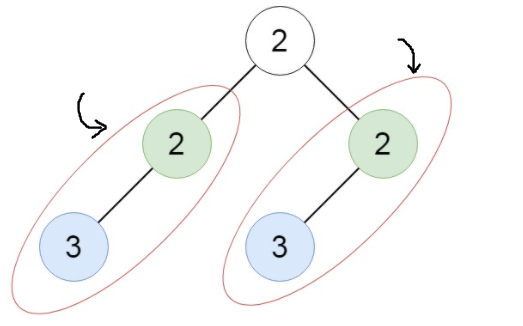# Duplicate Subtrees

Posted: 5 Dec, 2020
Difficulty: Moderate

## PROBLEM STATEMENT

#### Two subtrees are duplicate if and only if they have the same structure with the same node values.

##### For example:
``````In the below binary tree :
````````````The duplicate subtrees are {{2, 3}, {3}} and we have to just return the root values of each duplicate subtree, so the output is {2, 3}.
``````
##### Input Format:
``````The first line contains an integer 'T' which denotes the number of test cases or queries to be run. Then the test cases are as follows.

The only line of each test case contains elements in the level order form. The line consists of values of nodes separated by a single space. In case a node is null, we take -1 in its place. So -1 would not be a part of the tree nodes.

For example, the input for the tree depicted in the below image will be:
````````````For example taking a tree:

1
2 3
4 -1 5 6
-1 7 -1 -1 -1 -1
-1 -1
``````

#### Explanation :

``````Level 1 :
The root node of the tree is 1

Level 2 :
Left child of 1 = 2
Right child of 1 = 3

Level 3 :
Left child of 2 = 4
Right child of 2 = null (-1)
Left child of 3 = 5
Right child of 3 = 6

Level 4 :
Left child of 4 = null (-1)
Right child of 4 = 7
Left child of 5 = null (-1)
Right child of 5 = null (-1)
Left child of 6 = null (-1)
Right child of 6 = null (-1)

Level 5 :
Left child of 7 = null (-1)
Right child of 7 = null (-1)

The first not-null node(of the previous level) is treated as the parent of the first two nodes of the current level. The second not-null node (of the previous level) is treated as the parent node for the next two nodes of the current level and so on.

The input ends when all nodes at the last level are null(-1).
``````
##### Note :
``````The above format was just to provide clarity on how the input is formed for a given tree.
The sequence will be put together in a single line separated by a single space. Hence, for the above-depicted tree, the input will be given as:

1 2 3 4 -1 5 6 -1 7 -1 -1 -1 -1 -1 -1
``````
##### Output Format:
``````For each test case, print space-separated root node value of all the duplicate subtrees. If no duplicate subtree is present in the binary tree print ‘-1’. The order of the list of node values does not matter.

Print the output for each test case in a separate line.
``````
##### Note:
``````You don’t need to print anything; it has already been taken care of.
``````
##### Constraints:
``````1 <= T <= 5
1 <= N <= 100
1 <= val <=10^5

Time Limit: 1 sec
``````Approach 1

Our intuition here is to keep track of all the subtrees which are coming out to be a duplicate one. So we store the visited subtrees of the given binary tree with the help of hashing. And to traverse the tree, here we are taking in-order traversal of the given binary tree, where this recursive function returns the serialization of the subtrees in the form of a string in which we are enclosing the node values of the subtrees within “()” parentheses. At each node, we will record the result in our HashMap, and analyze the HashMap later to determine duplicate subtrees.

Steps are as follows:

• Firstly we use the inorder traversal technique to traverse the given binary tree.
• Now we store the inorder traversals of all the subtrees of the given binary tree into the HashMap.
• Since simple inorder traversal cannot uniquely identify a tree, we use symbols like ‘(‘ and ‘)’ to represent a complete node So that roots with value ‘1’ and ‘2’ can differ from the root “12”.
• We pass the HashMap as an argument to the helper function which recursively calculates the inorder traversal string of the subtrees, and simultaneously it increases its count in HashMap.
• If any string gets repeated, then it will imply the duplication of the subtree rooted at that node, so we push that root node in the final result and return the array/list of these node values.